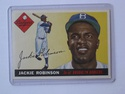## Distributions and Variability

Type of Unit: Project

# Prior Knowledge

Students should be able to:

• Represent and interpret data using a line plot.
• Understand other visual representations of data.

# Lesson Flow

Students begin the unit by discussing what constitutes a statistical question. In order to answer statistical questions, data must be gathered in a consistent and accurate manner and then analyzed using appropriate tools.

Students learn different tools for analyzing data, including:

• Measures of center: mean (average), median, mode
• Measures of spread: mean absolute deviation, lower and upper extremes, lower and upper quartile, interquartile range
• Visual representations: line plot, box plot, histogram

These tools are compared and contrasted to better understand the benefits and limitations of each. Analyzing different data sets using these tools will develop an understanding for which ones are the most appropriate to interpret the given data.

To demonstrate their understanding of the concepts, students will work on a project for the duration of the unit. The project will involve identifying an appropriate statistical question, collecting data, analyzing data, and presenting the results. It will serve as the final assessment.

Subject:
Mathematics, Statistics and Probability
Level:
Middle School
6
Tags:
Line Plots, Statistics, Box Plot, Center, Statistical Measures, Median, Range, Spread, 6th Grade Mathematics, Mode, Problem-solving, Line Plot, Estimation, Averages, Mean, 5 Number Summary, Variability, Five Number Summary, Histogram, Mean Absolute Deviation, Measurement, Data, Projects

Lesson 1

A Wrinkle in Time by Madeleine L'Engle (optional, for Reflection)

Lesson 2

Measuring tape (1 per measuring station)

Chalk (1 per measuring station)

Rulers (1 per pair or group)

Optional: Stopwatches (clock or watch with second hand will work) (1 per pair or group)

Unit 8 Distributions and Variability
•••••••••••••••••